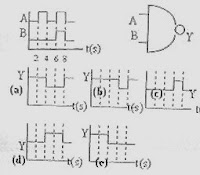## Sunday, April 29, 2007

### Five Kerala Engineering Entrance 2007 Questions (MCQ) from Electronics

Five questions from Electronics and three questions from Communication Systems were included in the Kerala Engineering Entrance 2007 (KEAM) question paper. Here are the questions from Electronics:

(1) The real time variation of input signals A and B are as shown below. If the inputs are fed in to NAND gate, then select the output signal from the followingThe NAND gate will give low output when all inputs are high. The output will be high in all other cases. The inputs A and B are high during the interval from 6 seconds to 8 seconds only. So, the output must be low during this interval only. This is indicated in option (b).

(2) In a common emitter amplifier, the current gain is 62. The collector resistance and the input resistance are 5 kΩ and 500 ohms respectively. If the input voltage is 0.01 V, the output voltage is

(a) 0.62 V (b) 6.2 V (c) 62 V (d) 620 V (e) 0.01 V

The collector current is given by Ic = βIB with usual notations.

But, the base current IB = Vi/Ri where Vi and Ri are the base voltage (input voltage) and input resistance respectively. Therefore, IB = 0.01/ 500 = 2 ×10–5 A.

The corresponding collector current is βIB = 62×2 ×10–5 A = 1.24×10–3 A

The output voltage is the voltage drop across the collector resistance (output resistance) Ro.

So, output voltage = IcRo = 1.24×10–3×5000 = 6.2 V.

[If you remember the simple expression for the voltage gain of a low frequency common emitter amplifier, the above result can be arrived at in a shorter time:

Voltage gain Av = βRo/Ri = 62×5000/500 = 620. Therefore output voltage = 620×0.01V = 6.2 V].

(3) The current gain of a transistor in common base mode is 0.995. The current gain of the same transistor in common emitter mode is

(a) 197 (b) 201 (c) 198 (d) 202 (e) 199

The expression for the current gain in common emitter mode is β = α /(1–α) where α is the current gain in common base mode.

Therefore, β = 0.995 /(1–0.995) = 199.

(4) When the forward bias voltage of a diode is changed from 0.6 V to 0.7 V, the current changes from 5 mA to 15 mA. The forward bias resistance is

(a) 0.01 Ω (b) ) 0.1 Ω (c) ) 10 Ω (d) ) 100 Ω (e) ) 0.2 Ω

Forward resistance, R = (Change in forward biasing voltage) /(Change in forward current) = (0.1 V)/ 10 mA = 0.1/(10×10–3) Ω = 10 Ω.

(5) The energy gap between conduction band and the valence band of a material is of the order of 0.7 eV. Then it is

(a) an insulator (b) ) a conductor (c) a semiconductor

(d) an alloy (e) a superconductor

If the energy gap between the conduction band and the valence band is less than 3 eV, the substance is a semiconductor. So, the correct option is (c).